# BITSAT Maths Test - 2

## 45 Questions MCQ Test BITSAT Subject Wise & Full Length Mock Tests | BITSAT Maths Test - 2

Description
This mock test of BITSAT Maths Test - 2 for JEE helps you for every JEE entrance exam. This contains 45 Multiple Choice Questions for JEE BITSAT Maths Test - 2 (mcq) to study with solutions a complete question bank. The solved questions answers in this BITSAT Maths Test - 2 quiz give you a good mix of easy questions and tough questions. JEE students definitely take this BITSAT Maths Test - 2 exercise for a better result in the exam. You can find other BITSAT Maths Test - 2 extra questions, long questions & short questions for JEE on EduRev as well by searching above.
QUESTION: 1

### The area (in square units) of the region enclosed by the curves y = x2 and y = x3 is

Solution: By solving equations 1and 2 we can get 0,1as values for x which can be the limits and by subtracting y=x^2 from x^3 and integrating the result within the limits 0 to 1 we can get area = 1\12
QUESTION: 2

### In the expansion of (y1/6 - y-1/3)9 the term independent of y is :

Solution: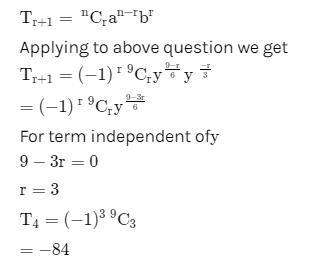QUESTION: 3

### Equation of the diameter of the circle x2 + y2 - 6x + 2y = 0 which passes thro' the origin is

Solution:
QUESTION: 4

If the two circles 2x2 + 2y2 -3x + 6y + k = 0 and x2 + y2 - 4x + 10y + 16 = 0 cut orthogonally, then the value of k is

Solution:
QUESTION: 5

If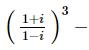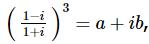then a and b are

Solution:
QUESTION: 6

The order and degree of differential equation √(dy/dx) - 4 (dy/ dx) - 7x = 0 are

Solution:

√(dy/ dx)- 4 (dy/dx) -7x = 0
or √(dy/ dx) = 4 (dy/dx) + 7x
Squaring both side
dy/ dx = [4 (dy/dx ) + 7x]2
or dy dx = 16 ( dy/dx )2 + 49x2 + 56 dy/ dx x
∴ order = 1
degree = 2

QUESTION: 7

If x dy = y(dx + y dy), y > 0 and y (1) = 1, then y (-3) is equal to

Solution: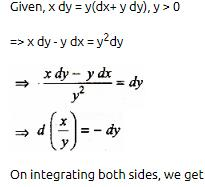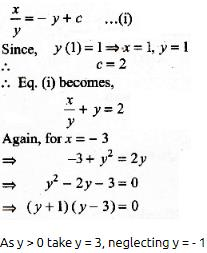QUESTION: 8

Solution of the differential equation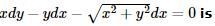Solution:
QUESTION: 9

If x=a[(cost)+(log tan(t/2))], y=a sint, (dy/dx)=

Solution:
QUESTION: 10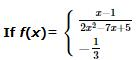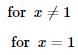then  = f '(1)

Solution:
QUESTION: 11

1/2! - 1/3! + 1/4! - 1/5! + ....... equals

Solution:
QUESTION: 12

The length of the shadow of a rod inclined at 10o to the vertical towards the sun is 2.05 metres when the elevation of the sun is 38o.The length of the rod is

Solution:
QUESTION: 13

The area of the region bounded by the curve y = x - x2 between x = 0 and x = 1 is

Solution:
QUESTION: 14

The product of the perpendicular, drawn from any point on a hyperbola to its asymptotes is

Solution:
QUESTION: 15

The area bounded by the curve x = at2, y = 2at and the X-axis is 1 ≤ t ≤ 3 is

Solution:
QUESTION: 16

The sum of first 50 terms of the series cot⁻13 + cot⁻1 7 + cot⁻1 13 + cot⁻1 21 + ... is

Solution:
QUESTION: 17

The domain of the function f(x) = √(2 - 2x - x2) is

Solution:
QUESTION: 18

The difference of an integer and its cube is divisible by

Solution:
QUESTION: 19

If A and B are two matrices such that AB = B and BA = A, then A2 + B2 is equal to

Solution:
QUESTION: 20

if A is a 3 x 3 matrix and B is its adjoint matrix. If ∣B∣ = 64, then ∣A∣ =

Solution:
QUESTION: 21

The strength of a beam varies as the product of its breadth b and square of its depth d. A beam cut out of a circular log of radius r would be strong when

Solution: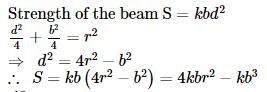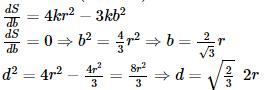QUESTION: 22

Which of the following is correct

Solution:
QUESTION: 23

Equation x2+7xy+3y2+8x+14y+λ=0 represents a pair of straight lines, value of λ is

Solution:
QUESTION: 24

The pole of the line lx+my+n=0 w.r.t. the parabloa y2 =4ax

Solution:
QUESTION: 25

The latus rectum of the parabola y2 = 5x + 4y + 1 is

Solution:

y2 = 5x + 4y + 1
or y2 - 4y = 5x + 1
or y2 - 2.2.y + (2)2 - (2)2 = 5x + 1
or (y - 2)2 = 5x + 5
or (y - 2)2 = 5(x + 1)
Length of the latus rectum = 5

QUESTION: 26

How many total words can be formed from the letters of the word INSURANCE in which vowels are always together?

Solution:
QUESTION: 27

A polygon has 44 diagonals. The number of its sides is

Solution: The number of diagonals for n sided polygon = [n(n-3)]/2 . therefore, => 44 = [n(n-3)]/2 . => n^2 - 3n - 88 = 0 . => (n+8)(n-11) =0. => n = -8 or 11. Neglect n = -8. Therefore, => n = 11 . therefore, the no. of sides = 11 Hence, correct answer is (B).
QUESTION: 28

A committee consists of 9 experts from three institutions A, B and C, of which 2 are from A, 3 from B and 4 from C. If three experts resign, then the probability that they belong to different institutions is

Solution:
QUESTION: 29

A single letter is selected at random from the word "PROBABILITY". The probability that it is a vowel is

Solution:
QUESTION: 30

If the area of a Δ A B C be λ then a2 sin 2B + b2 sin 2A is equal to

Solution: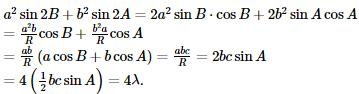QUESTION: 31

In a Δ A B C , a = 1 and the perimeter is six times the AM of the sines of the angles. The measure of ∠ A is

Solution: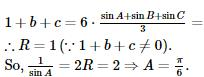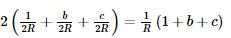QUESTION: 32

If α, β are the roots of the equation x2- 2x + 2 = 0, then the value of α2 + β2 is

Solution:
QUESTION: 33

The sum of an infinite G.P. is 3. The sum of the series formed by squaring its terms is also 3. The series is

Solution:
QUESTION: 34

The 5th term of a G.P. is 2, then the product of its first 9 term is

Solution:
QUESTION: 35

If f : R → R is given as f (x) = |x| and A = {x ∈ R : x > o}, then f⁻1(A) =

Solution:
QUESTION: 36

If f (x) = {2x - 3, x ≤ 2} {x, x < 2} then f (1) is equal to

Solution:
QUESTION: 37

The equation line passing through the point P(1,2) whose portion cut by axes is bisected at P, is

Solution: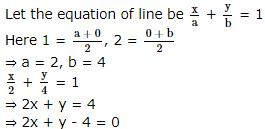QUESTION: 38

The equation of the common tangent to the curves y2=8x and xy=-1 is :

Solution:
QUESTION: 39

The radius of the circle in which the sphere x2 + y2 + z2 + 2x - 2y - 4z - 19 = 0 is cut by the plane x + 2y + 2z + 7 = 0, is

Solution: Radius of sphere is=5 then perpen dicular dice Frome plane=4 now radius of circle r,sqr=25_16=9so r=3
QUESTION: 40

The acute angle between the planes 2x-y+z=6 and x+y+2z=3 is

Solution:
QUESTION: 41

If 0≤x≤π and 81sin2x + 81 cos2x = 30, then x is equal to

Solution:
QUESTION: 42

If 1 + sin x + sin 2 x + … ∞ = 4 + 2 √ 3 , 0 < x < π , x ≠ π/2 then x =

Solution:
QUESTION: 43

(secA+tanA-1)(secA-tanA+1)-2 tanA=

Solution:
QUESTION: 44

The value of b such that the scalar product of the vector î+ĵ+k̂ with the unit vector parallel to the sum of the vectors 2î + 4ĵ - 5k̂ and bî+ 2ĵ + 3k̂ is one is

Solution:

Parallel vector =(2+b)i+6j−2k

Unit vector = (2+b)i+6j−2k / (b2+4b+44)1/2

According to the question,

1 = (2+b)+6−2/b2+4b+44

⇒b2+4b+44=b2+12b+36

⇒8b=8⇒b=1

QUESTION: 45

The angle between the vectors 3i+j+2k and 2i-2j+4k is

Solution: